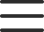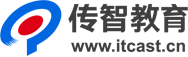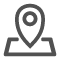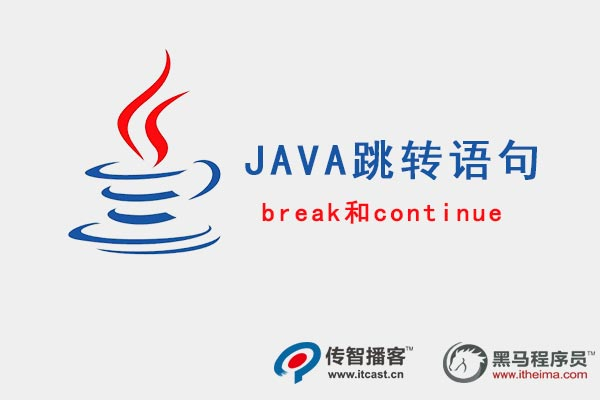# Java 跳转语句break和continue的用法

JAVA跳转语句用于实现循环执行过程中程序流程的跳转,在Java中的跳转语句有 break语句和 continue语句。接下传智播客小编将来分别进行详细地讲解。

1. break语句

public class Example{
public static void main(String[] args){
int x=1;
while (x<=4){
System.out. println("x="+x);
If (x==3){
break;
}
x++;
}
}
}

public class Example{
public static void main(String[] args){
int x= i,j;
intcast: for (i=1;i<=9;i++) {
for (j=1;j<=i;j++) {
if (i>4) {
break intcast;
}
System.out.print("*");
}
System.out.print("\n");
}
}

*
**
***
****2、continue语句
continue语句用在循环语句中,它的作用是终止本次循环,执行下一次循环。接下来对1~100之内的奇数求和。

public class Example{
public static void main(String[] args){
int sum=0;           //定义变量sum，用于记住和
for (int i=1;i<=100;i++) {
if (i %2==0) {     //i是一个偶数，不累加
continue;    // 结束本次循环
}
sum +=i;          //实现sum和i的累加
}
System.out.println("sum"=+sum);
}

python难学吗